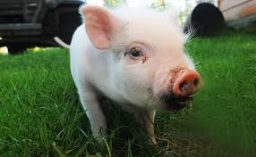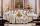# The piglet

The weight of the piglet grew regularly by five kilograms for four months. Determine the proportion by weight of the piglet in each month, if the first weighed 35 kg.

Result

r1 =  8:7
r2 =  9:8
r3 =  10:9
r4 =  11:10

#### Solution:

$m_{0}=35 \ \text{kg} \ \\ d=5 \ \text{kg} \ \\ m_{1}=m_{0}+d=35+5=40 \ \text{kg} \ \\ \ \\ r_{1}=m_{1}/m_{0}=40/35 \doteq \dfrac{ 8 }{ 7 } \doteq 1.1429≈ 1.1429 \doteq 8:7$
$m_{2}=m_{1}+d=40+5=45 \ \text{kg} \ \\ r_{2}=m_{2}/m_{1}=45/40=\dfrac{ 9 }{ 8 }=1.125=9:8$
$m_{3}=m_{2}+d=45+5=50 \ \text{kg} \ \\ r_{3}=m_{3}/m_{2}=50/45 \doteq \dfrac{ 10 }{ 9 } \doteq 1.1111≈ 1.1111 \doteq 10:9$
$m_{4}=m_{3}+d=50+5=55 \ \text{kg} \ \\ r_{4}=m_{4}/m_{3}=55/50=\dfrac{ 11 }{ 10 }=1.1=11:10$Our examples were largely sent or created by pupils and students themselves. Therefore, we would be pleased if you could send us any errors you found, spelling mistakes, or rephasing the example. Thank you!

Leave us a comment of this math problem and its solution (i.e. if it is still somewhat unclear...):Be the first to comment!Tips to related online calculators
Check out our ratio calculator.

## Next similar math problems:

1. Heptagonal pyramidA hardwood for a column is in the form of a frustum of a regular heptagonal pyramid. The lower base edge is 18 cm and the upper base of 14 cm. The altitude is 30 cm. Determine the weight in kg if the density of the wood is 10 grams/cm3.
2. The copper wireThe copper wire bundle with a diameter of 2.8mm has a weight of 5kg. How many meters of wire is bundled if 1m3 of copper weighs 8930kg?
3. Friction coefficientWhat is the weight of a car when it moves on a horizontal road at a speed of v = 50 km/h at engine power P = 7 kW? The friction coefficient is 0.07
4. Iron densityCalculate the weight of a 2 m long rail pipe with an internal diameter of 10 cm and a wall thickness of 3 mm. The iron density is p = 7.8 g/cm3.
5. Density of the concreteFind the density of the concrete of the cuboid-shaped column has dimensions of 20 x 20 cm x 2 m if the weight of the column is 200 kg.
6. A carA car weighing 1.05 tonnes driving at the maximum allowed speed in the village (50 km/h) hit a solid concrete bulkhead. Calculate height it would have to fall on the concrete surface to make the impact intensity the same as in the first case!
7. Copper sheetThe copper plate has a length of 1 m, width 94 cm and weighs 9 kg. What is the plate thickness, if 1 m3 weighs 8715 kg?
8. The water tankThe water tank has the shape of a sphere with a radius of 2 m. How many liters of water will fit in the tank? How many kilograms of paint do we need to paint the tank, if we paint with 1 kg of paint 10 m2?
9. Iron poleWhat is the mass of pole with the shape of a regular quadrilateral prism with a length of 1 m and a cross-sectional side length of a = 4.5 cm make from iron with density ρ = 7800 kg/m³?
10. Children poolThe bottom of the children's pool is a regular hexagon with a = 60 cm side. The distance of opposing sides is 104 cm, the height of the pool is 45 cm. A) How many liters of water can fit into the pool? B) The pool is made of a double layer of plastic film
11. Orlík hydroelectric plantThe Orlík hydroelectric power plant, built in 1954-1961, consists of four Kaplan turbines. For each of them, the water with a flow rate of Q = 150 m3/s is supplied with a flow rate of h = 70.5 m at full power. a) What is the total installed power of the
12. Cu thiefThe thief stole 121 meters copper wire with cross-section area of 103 mm2. Calculate how much money gets in the scrap redemption, if redeemed copper for 4.6 eur/kg? The density of copper is 8.96 t/m3.
13. PumpWhat power has a pump output to move 4853 hl of water to a height of 31 m for 8 hours?
14. Angled cyclist turnThe cyclist passes through a curve with a radius of 20 m at 25 km/h. How much angle does it have to bend from the vertical inward to the turn?
15. Car crashOn the road, with a maximum permitted speed of 60 km/h, there was a car crash. From the length of the vehicle's braking distance, which was 40 m, the police investigated whether the driver did not exceed that speed. What is the conclusion of the police, a
16. Water levelHow high is the water in the swimming pool with dimensions of 37m in length and 15m in width, if an inlet valve is opened for 10 hours flowing 12 liters of water per second?
17. 3d printer3D printing ABS filament with diameter 1.75 mm has density 1.04 g/cm3. Find the length of m = 5 kg spool filament. (how to calculate length)